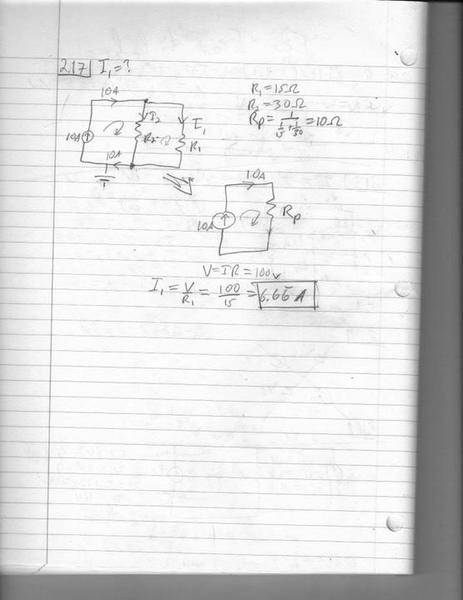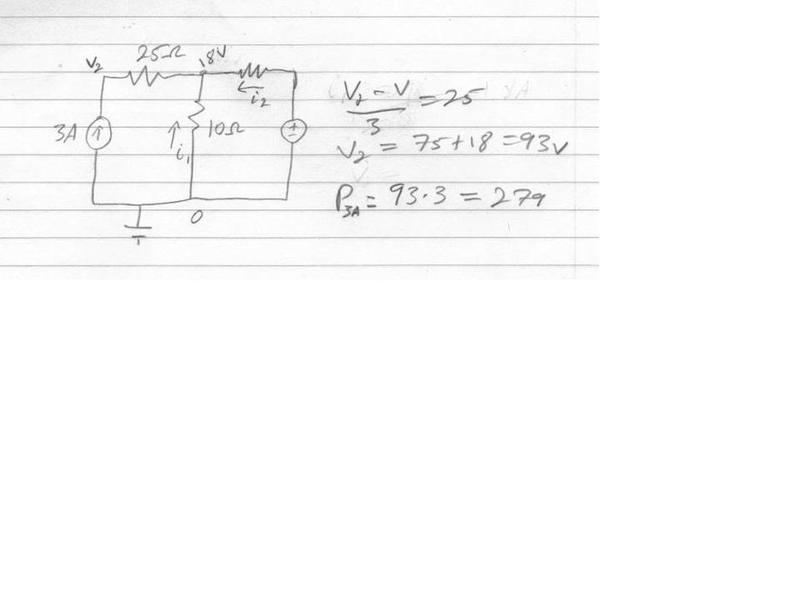# Can someone explain my answer to me? I want to understand it.

• frozenguy
In summary, current flows through the resistor and the voltage drop over the resistor is what creates the 100 volts on the opposite side.f

## Homework Statement

Hi physics forums, thanks for responding if you doUse Ohm's law and Kirchhoff's current law to determine the current I1 in the circuit of Figure--

## The Attempt at a Solution

So I'm trying to fully understand what is going on here..

Besides not getting where the voltage comes from without a voltage source; where is the 100volts? Its the drop over the equivalent resister Rp, I know that. So I guess there is 0 volt on the ground side (-?) of the current source and 100 volts on the opposite (+?) side.

I used 100 volt to find the current through R1, but its 100 volts through R2 also? How is it 100 volts through both?You have a current source in parallel with a resistance (R1 or R2 or RP) which can be modeled as a voltage source in series with a resistance (maybe not learned that yet? you don't need to do it to calculate the voltages anyway).

Either way, you can have a voltage with no current flowing (terminals of a battery) but you can never a current flowing with no voltage, thus to have a current source, there must be a voltage somewhere driving the current.

Ohms Law shows us this, in that for any current flowing through a resistor there will be a voltage V, as you have calculated.

Yes the (-) is 0 and the (+) is 100, as per Kirchoffs Voltage law around any of the loops.

Are you aware of the general rule that voltages in parallel are equal and current in series is the same everywhere?

You have a current source in parallel with a resistance (R1 or R2 or RP) which can be modeled as a voltage source in series with a resistance (maybe not learned that yet? you don't need to do it to calculate the voltages anyway).
Thevenin equivalent? We were lectured on it but I haven't studied it. And its after this section in the book.

Either way, you can have a voltage with no current flowing (terminals of a battery) but you can never a current flowing with no voltage, thus to have a current source, there must be a voltage somewhere driving the current.

Ohms Law shows us this, in that for any current flowing through a resistor there will be a voltage V, as you have calculated.

Yes the (-) is 0 and the (+) is 100, as per Kirchoffs Voltage law around any of the loops.

Are you aware of the general rule that voltages in parallel are equal and current in series is the same everywhere?

Isn't V=I1R1 the voltage drop over the resister? Meaning it has less voltage after it goes through? So how can the voltage drop be 100 for each if there is only 100 to begin with?

Did I do this right? Finding the power delivered by the current source?

My teacher says its negative, but I see positive voltage on the positive side of the current source, and its a positive current. So how does he get negative?